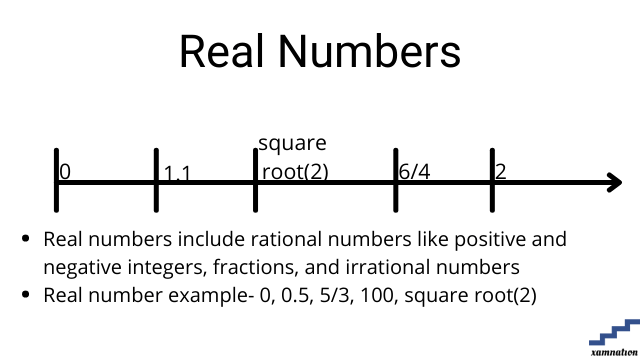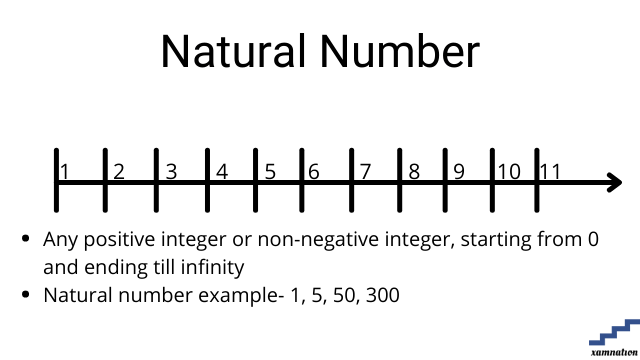# Maths for Grade 9 – Number System | what is real number | what is natural number | what is Fraction

Number system tutorial

Number system is system of representing numbers in mathematics. Students deal with various types of numbers for solving mathematics formulas and calculation, data processing and handling complex topics like algebra and geometry.

In this article, we have shared different types of numbers that are used in mathematics for grade 9 and above. This article can be used by all grades students, as the definition of these different types of numbers is same for all grades.

## What is Real Number?

### Definition of real number

• Real numbers include rational numbers like positive and negative integers, fractions, and irrational numbers
• Real number example- 0, 0.5, 5/3, 100, square root(2)### Examples of real number

• 0, 1.1, 3/2 are example of real numbers
• All integer, decimal, rational ,irrational numbers are real numbers

Practice question on real number

Can you find whether below mentioned numbers are real or not?

• 10
• 5
• 10/13
• -1.1
• -2/3
• 0

Send your solution of finding real numbers to info@xamnation.com , and know detailed answer of this problem.

## What is Natural number?

### Definition of natural number

• Any positive integer or non-negative integer, starting from 0 and ending till infinity
• Natural number example- 1, 5, 50, 300### Examples of natural number

• 5, 10, 500, 3500 are natural number
• 0, -5, -20, -45 are not natural number
• 6 , 1/3 are not natural number

Practice question on natural number

Can you find whether below mentioned numbers is natural number or not?

• 10
• 5
• -15
• 10/13
• -1.1

Send your solution of finding natural number to info@xamnation.com , and know detailed answer of this problem.

## What is Fraction

### Definition of Fraction

A fraction is a part of a whole or a given number. It is represented by p/q, where p and q are integer.

### Examples of Fraction

• 1/5, 2/3 are example of fraction
• Fraction can be more than 1, but cannot be less than 0Practice question on fraction

Can you find whether below mentioned numbers is fraction or not?

• 10
• 5
• -15
• 10/13
• -1.1

Send your solution of finding fraction to info@xamnation.com , and know detailed answer of this problem.

## Conclusion

In this article, we have seen how to find whether number is real number, natural number and fraction. We also saw detailed examples and practical exercises for number system for grade 9 mathematics.

Are you struck in number system problem or any mathematics topics for grade 9 and above?

You can reach out to us for your maths doubts and problem; our maths tutors will help you in solving your doubts and take one-one maths sessions online. Reach out to us, and our qualified maths tutors for grade 6 and above will be glad to help you.